5th Grade Operations
5th Grade Algebra
6th Grade Number Systems
6th Grade Statistics &
Probability
6th Grade Equations
100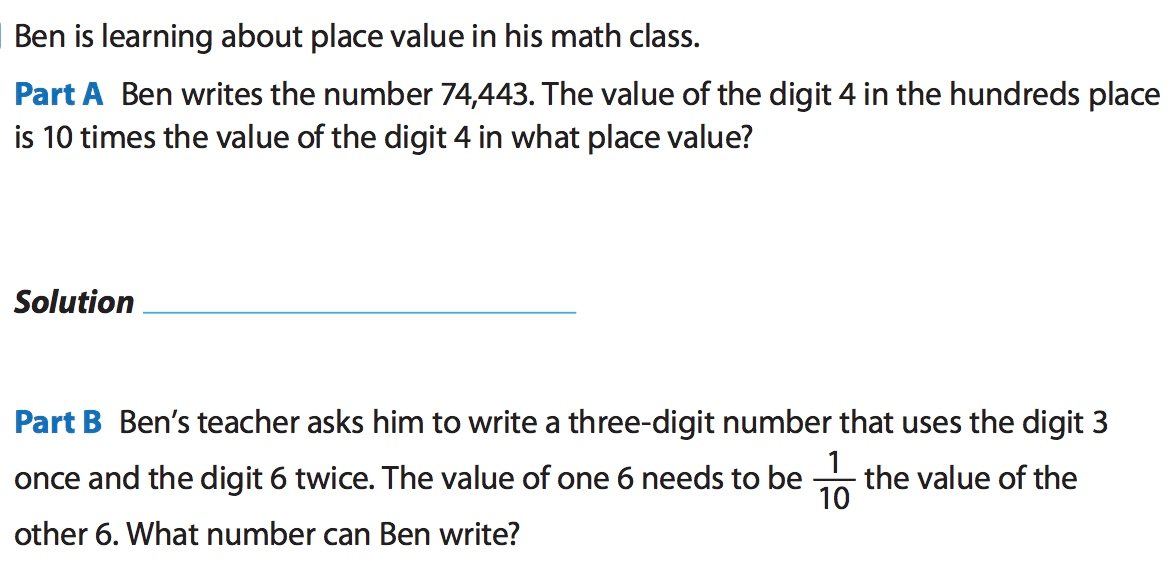What is A = tens place and B = 366 or 663?

100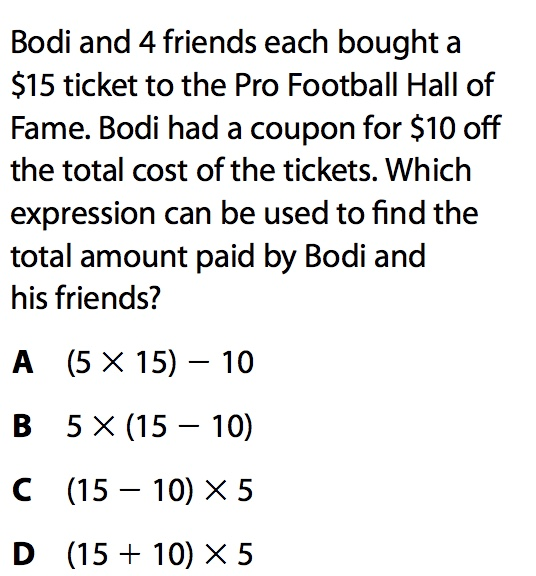What is A?

100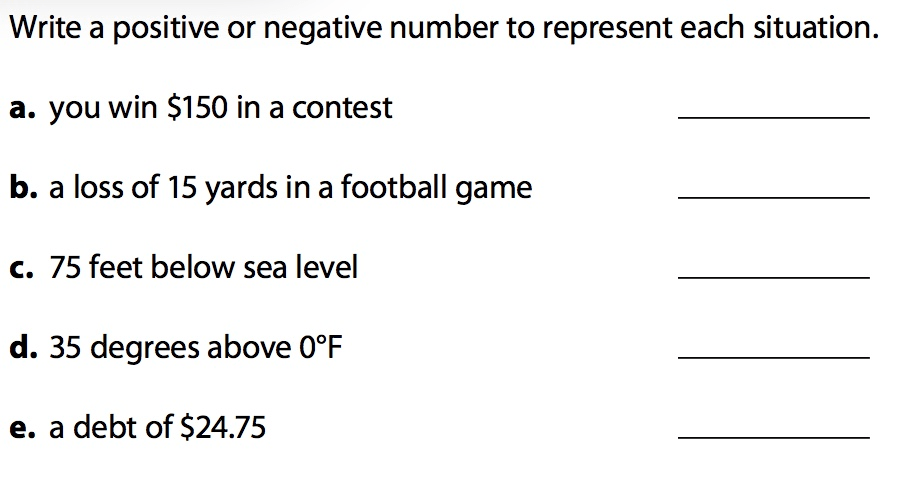100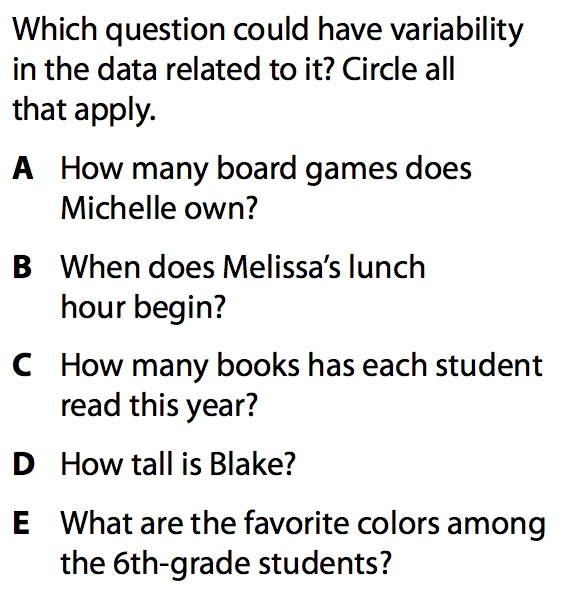What are C and E?

100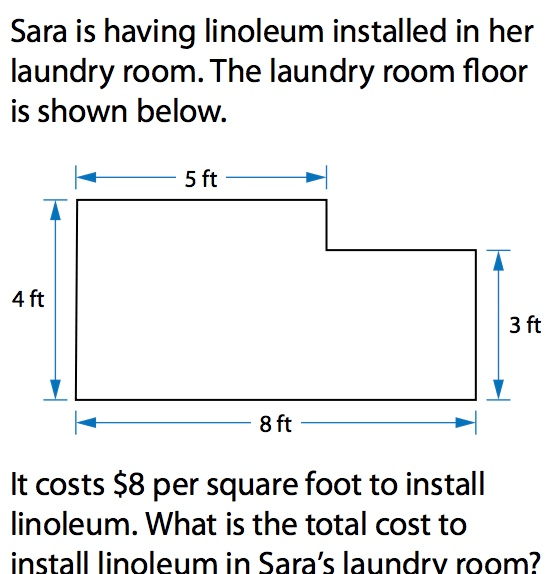What is \$216?

200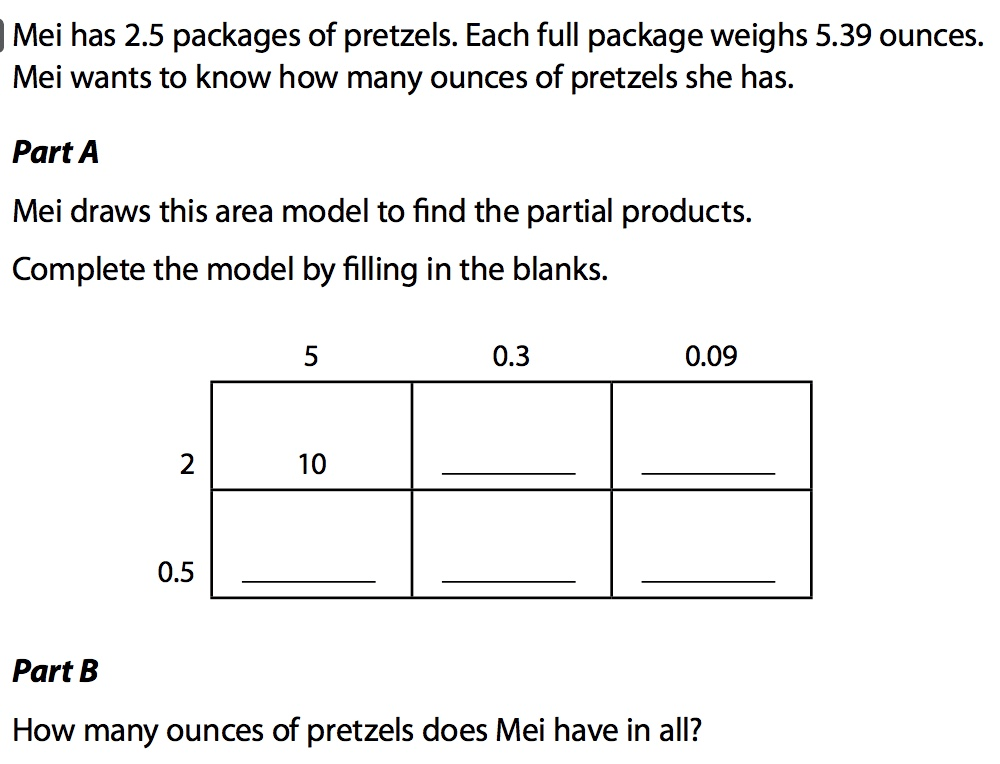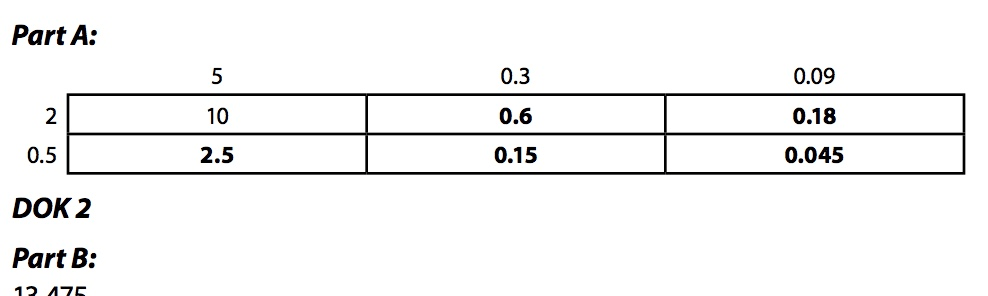200What are false, false, true, true?

200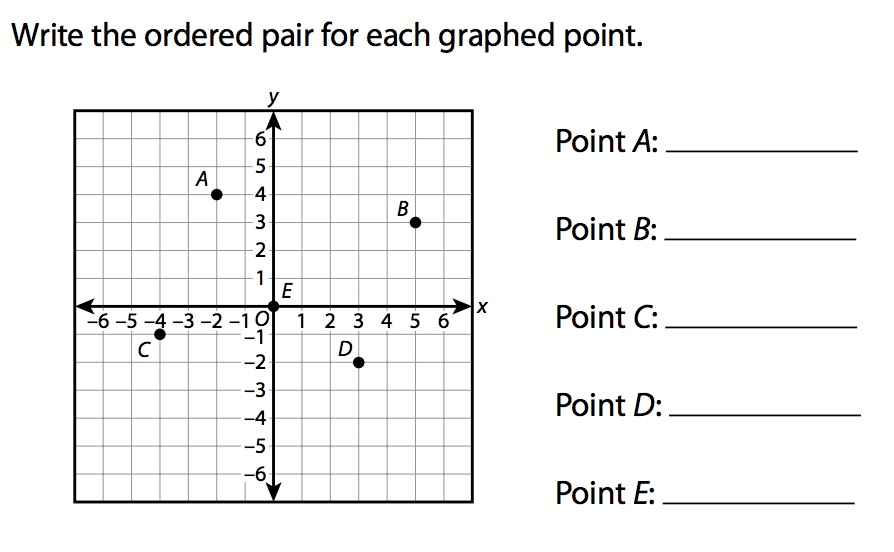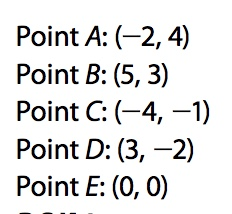200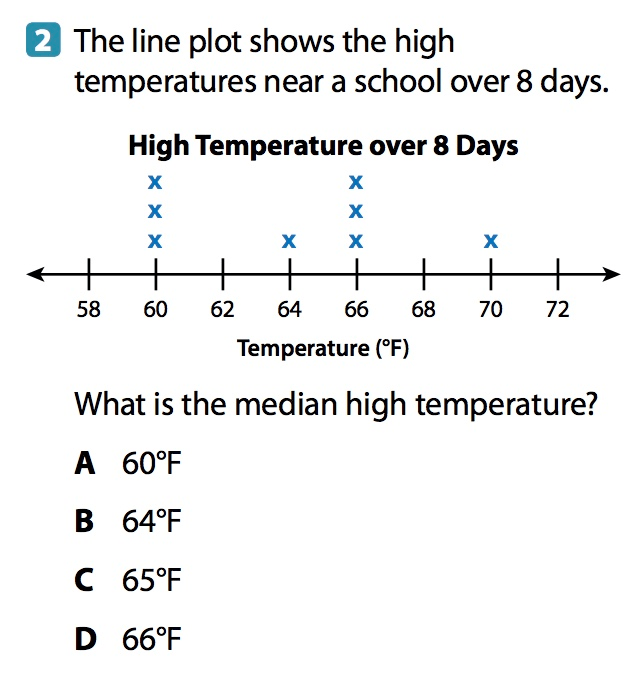What is C?

200What is B?

300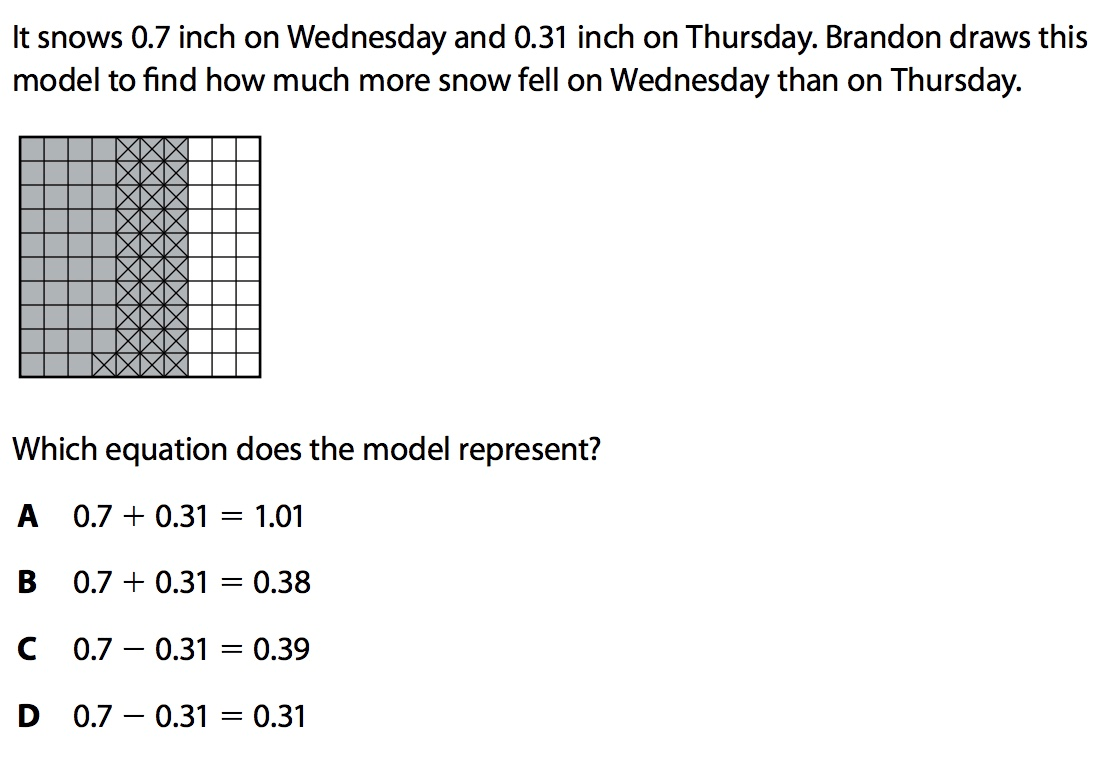What is C?

300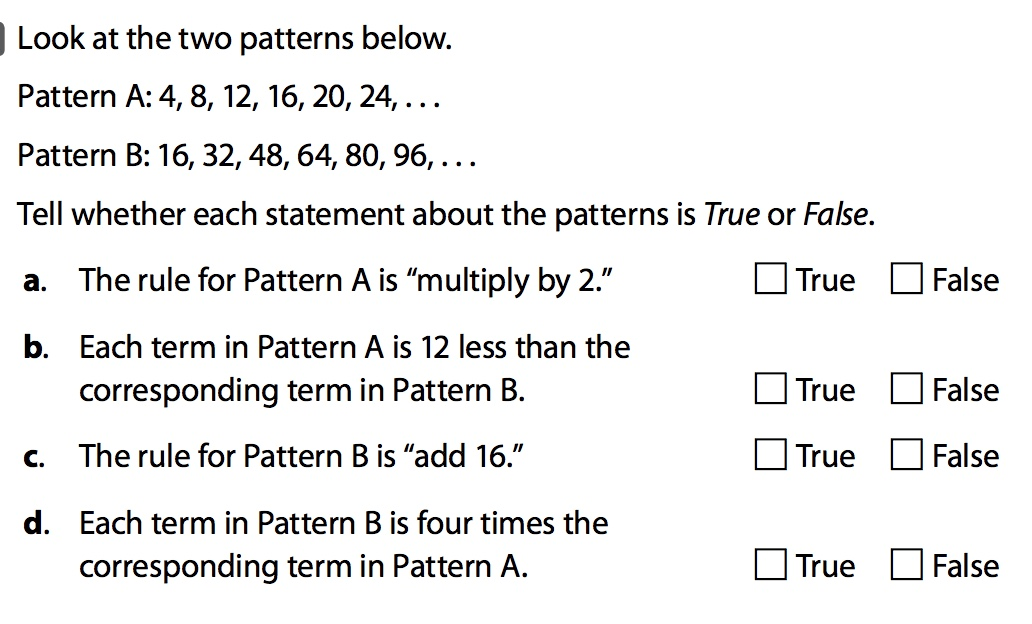What are false, false, true, true?

300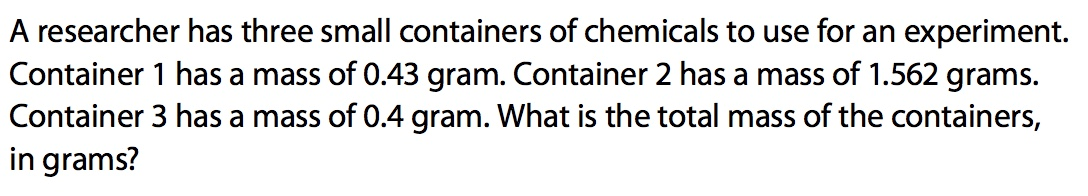What is 2.392?

300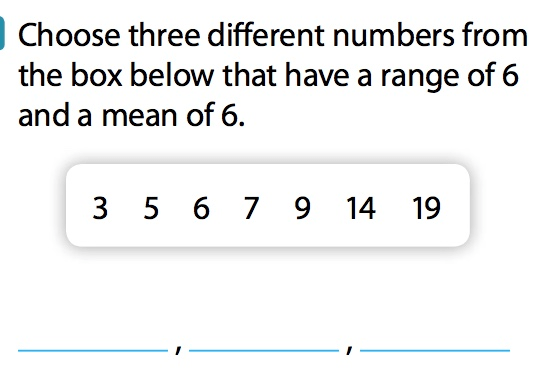What are 3, 6, and 9?

300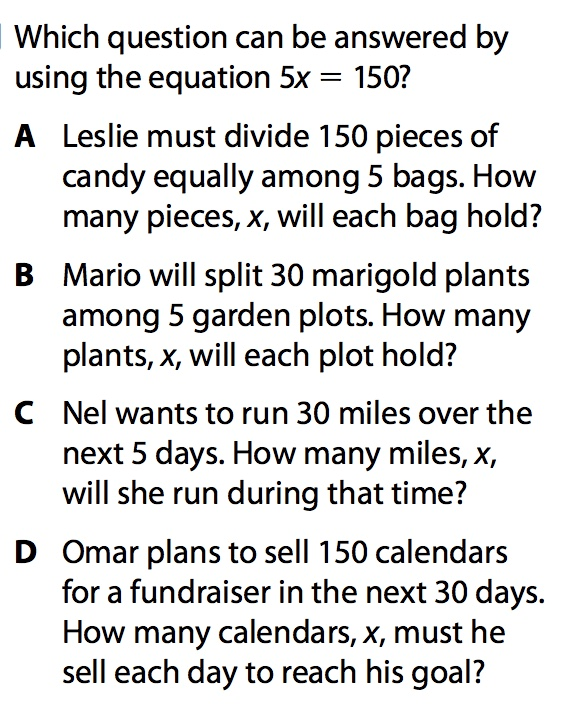What is A?

400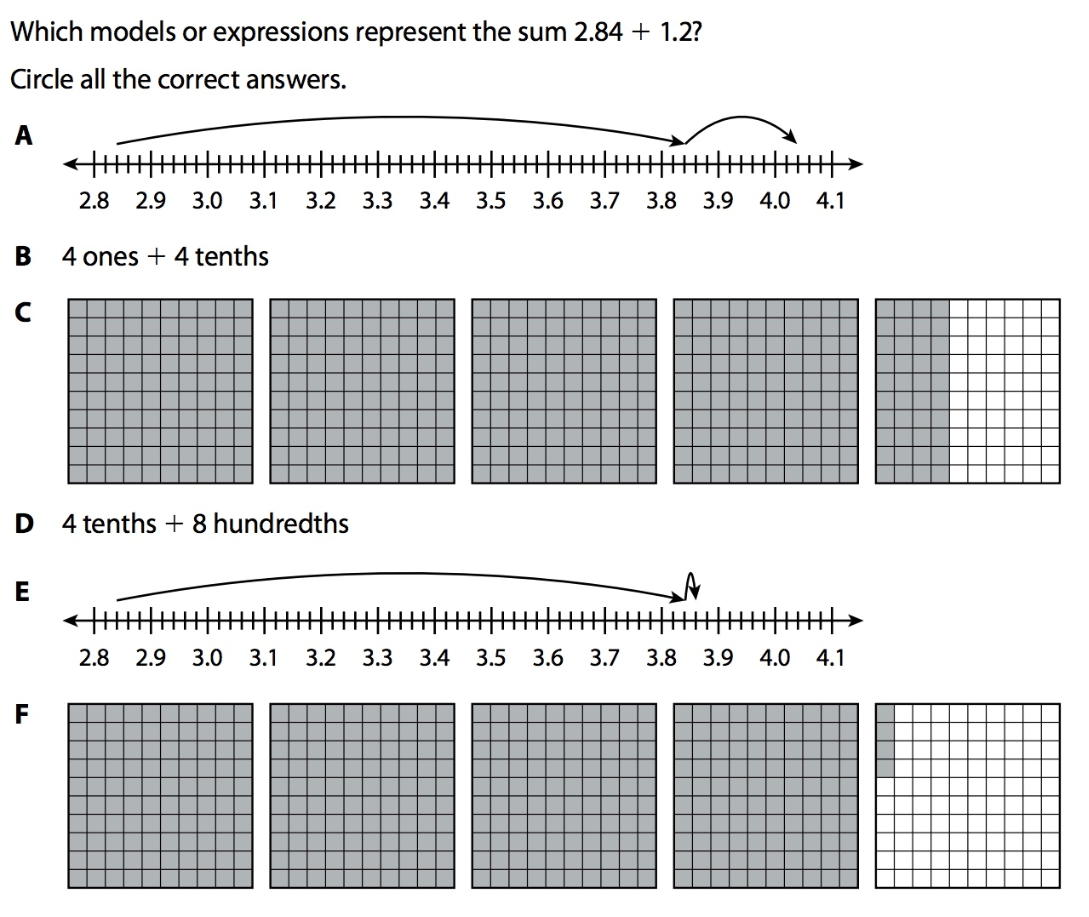What are A and F?

400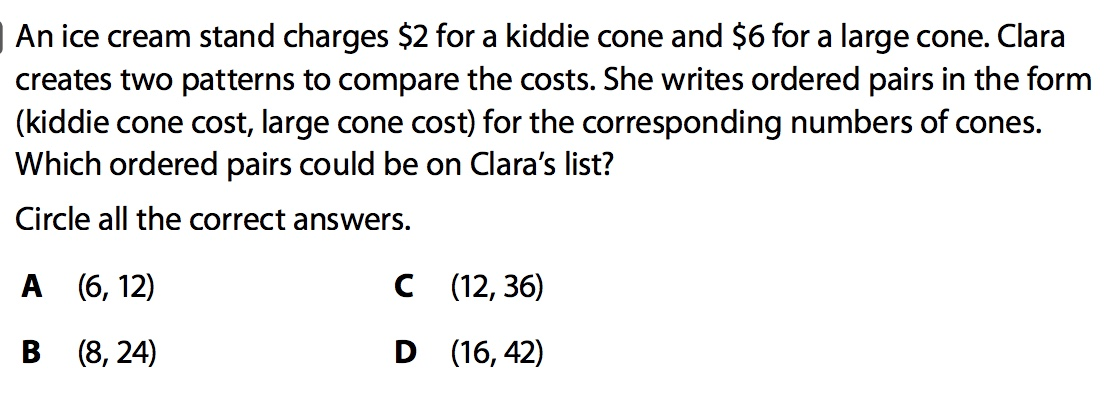What are B and C?

400What is C?

400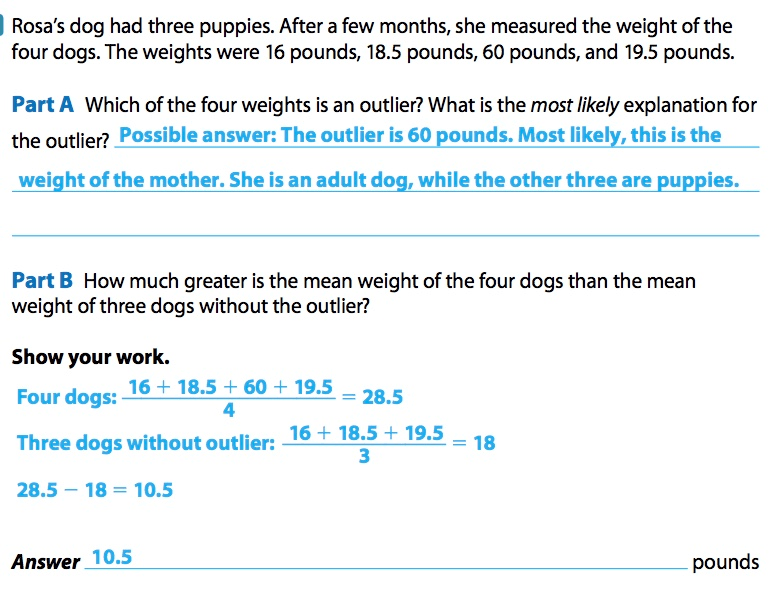400What is false, true, true, true?

500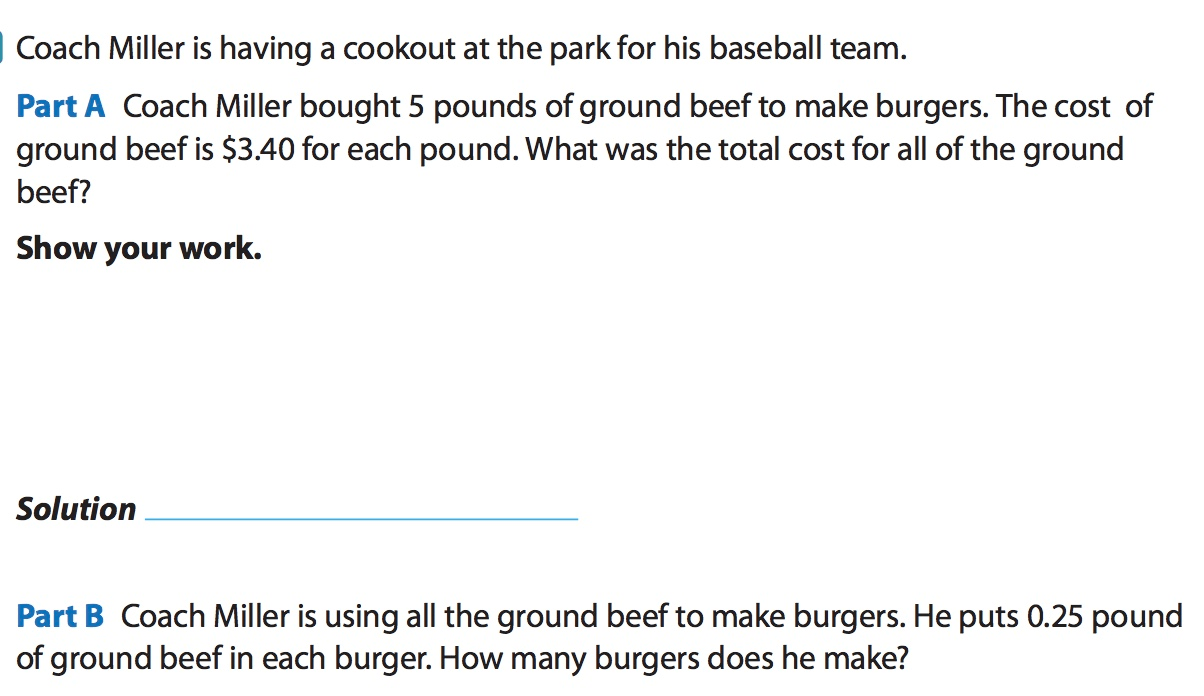What are \$17 and 20 burgers?

500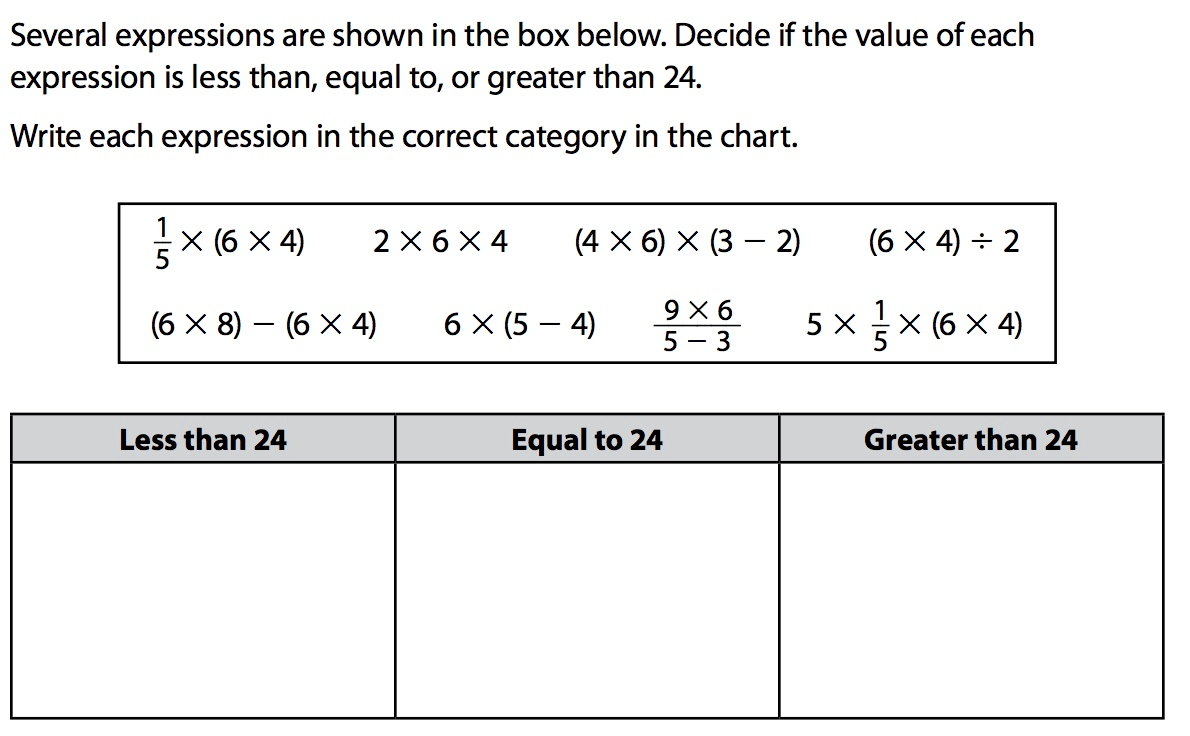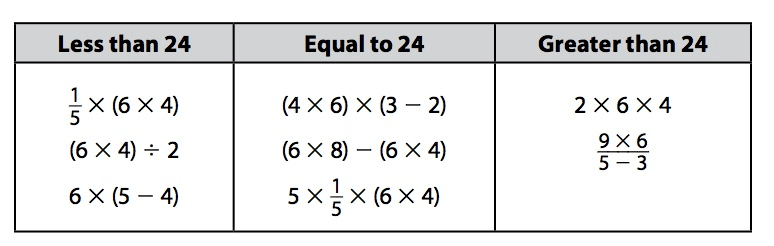500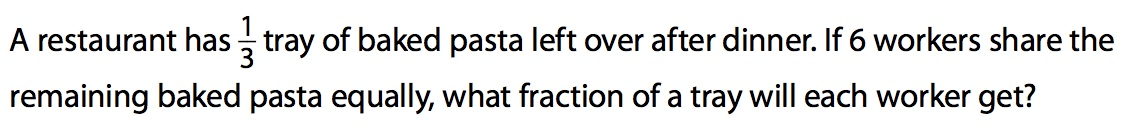What is 1/18?

500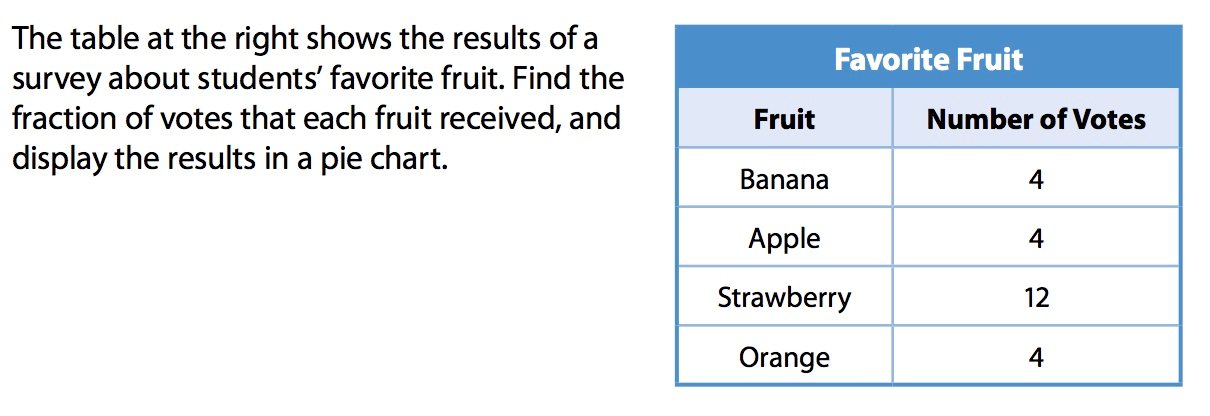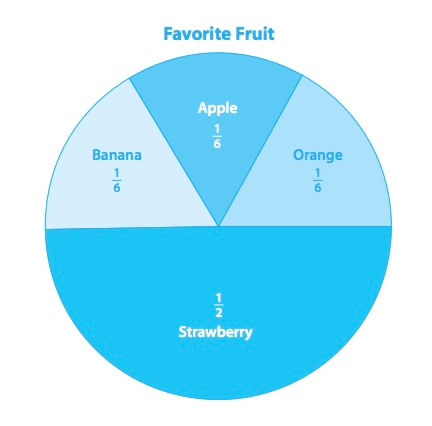500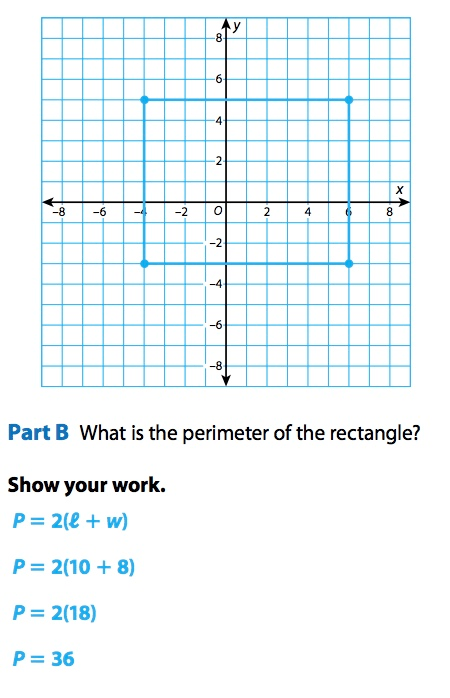Click to zoom